Before talking about the types of quadrilaterals, let us recall what a quadrilateral is. A quadrilateral is a polygon which has the following properties

• 4 vertices and 4 sides enclosing 4 angles
• The sum of all interior angles of a quadrilateral is 360 degrees
• We can also derive the sum of interior angle from the formula of polygon i.e. (n -2) × 180, where n is equal to the number of sides of the polygon

A quadrilateral, in general, has sides of different lengths and angles of different measures. However, squares, rectangles, etc. are special types of quadrilaterals with some of their sides and angles being equal. This is the reason that the area of quadrilateral depends on which type of quadrilateral it is. In this article, we will discuss the special types of quadrilaterals and their basic properties.

There are six basic types of quadrilaterals. They are:

1. Trapezium
2. Parallelogram
3. Rectangle
4. Rhombus
5. Square
6. Kite

### Trapezium

It is a quadrilateral with one pair of opposite parallel sides. In the trapezium, ABCD, side AB is parallel to side CD.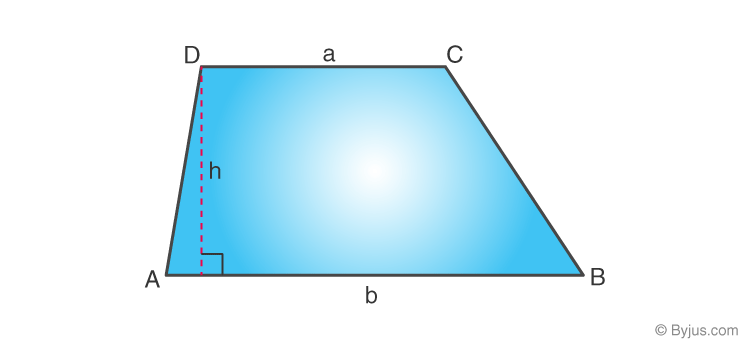### Parallelogram

It is a quadrilateral with two pairs of parallel sides. The opposite sides are parallel and equal in length. The opposite angles are equal in measure. In the parallelogram, ABCD, side AB is parallel to side CD and side AD is parallel to side BC.
Also, the two diagonals formed to intersect each other at the midpoints. As in the figure given below, E is the point where both the diagonals meet. So
Length AE = EC, & Length BE = ED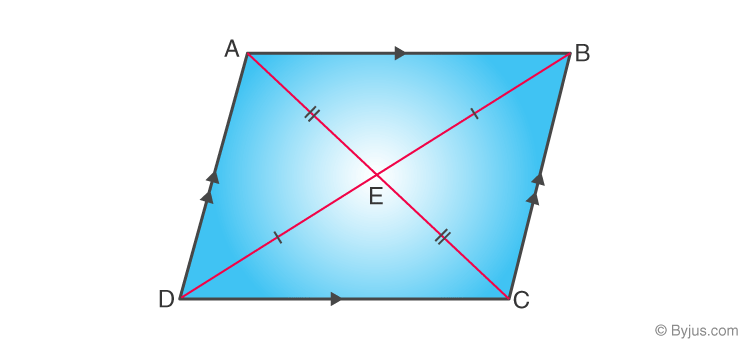### Rectangle

It is a quadrilateral with all the 4 angles of equal measure, that is, each of them is 90°. Both the pairs of opposite sides are parallel and equal in length.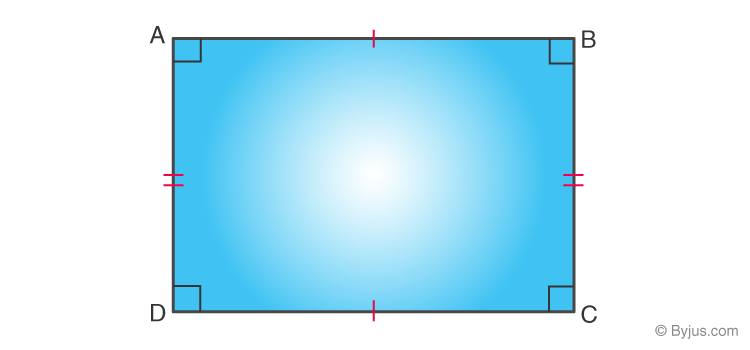### Rhombus

It is a quadrilateral with all four sides having equal lengths. The Opposite sides of a rhombus are parallel and opposite angles are equal.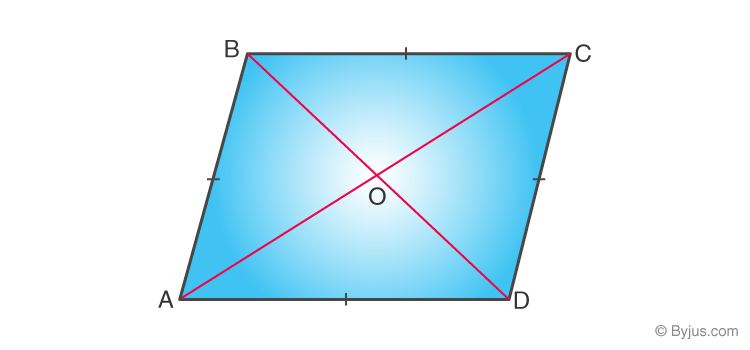### Square

It is a quadrilateral in which all the sides and angles are equal. Every angle is a right angle (i.e. 90° each). The pairs of opposite sides are parallel to each other.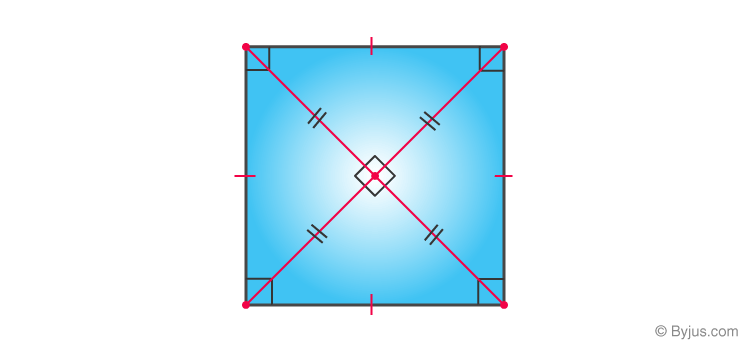### Kite

It is a quadrilateral that has 2 pairs of equal-length sides and these sides are adjacent to each other.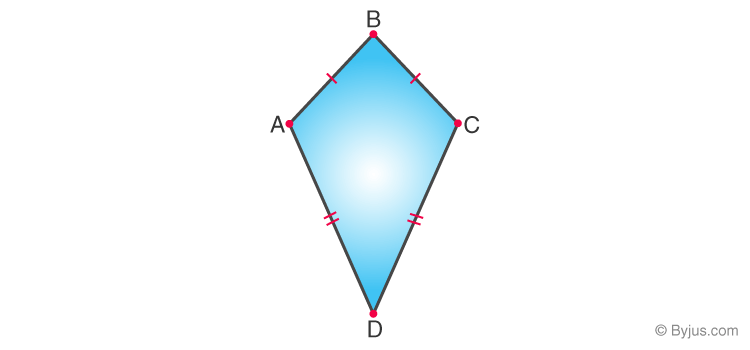• Square, rectangle, and rhombus are types of parallelograms.
• A square is a rectangle as well as a rhombus.
• The rectangle and rhombus are not a square.
• A parallelogram is a trapezium.
• A trapezium is not a parallelogram.
• Kite is not a parallelogram.

## Properties of Different Types of Quadrilaterals

The below table contains the properties of various types of quadrilaterals and their corresponding basic formulas.

 Type of Quadrilateral Properties Formulas Trapezium Only one pair of opposite sides are parallel Non-parallel sides are called legs Diagonals intersect each other The length of the mid-segment is equal to half the sum of the parallel bases Area of trapezium = (½) (a + b)h = (½) (Sum of two parallel sides) × HeightPerimeter = Sum of all the sides Parallelogram The opposite sides are parallel and equal The opposite angles are equal The sum of two consecutive angles is 180 degrees If any one of the angles is a right angle, then all the other angles will be right angles Diagonals bisect each other Each diagonal bisects the parallelogram into two congruent triangles Area = Base × Height Perimeter = Sum of all the side Rectangle The opposite sides are parallel and equal Each interior angle is a right angle The diagonals bisect each other and have the same length Area = Length × Breadth Perimeter = 2(Length + Breadth) Rhombus All sides of the rhombus are congruent, and the opposite sides are parallel Opposite angles of a rhombus are congruent Diagonals bisect each other at right angles Diagonals bisect the interior angles of a rhombus The sum of two adjacent angles is equal to 180 degrees Area = (½) (d1 × d2) = (½) (Product of the length of diagonals) Perimeter = 4(side length) Square All four sides of the square are equal, and the opposite sides of the square are parallel to each other The measure of each interior angle is 90 degrees The diagonals of the square are equal to each other and bisect each other at right angles Area  = (side)² Perimeter = 4(side) Kite Two pairs of adjacent sides are congruent One pair of opposite angles are equal  An axis of symmetry through one pair of opposite angles Two diagonals are not of the same length Diagonals intersect each other at right angles Area = (½) (d1 × d2) = (½) (Product of the length of diagonals) Perimeter = Sum of all the sides

## Solved Examples

Example 1:

If the perimeter of a square is 72 cm, then find its area.

Solution:

Let a be the side of a square.

Perimeter of a square = 4a

4a = 72 cm (given)

a = 72/4 = 18

Thus, side of the square = 18 cm

Area of the square = a² = (18)² = 324 cm²

Example 2:

The area of a trapezium is 180 cm², and its height is 9 cm. If one of the parallel sides is longer than the other by 6 cm, find the two parallel sides.

Solution:

Let x be the length of the shorter parallel side.

So, the length of the longer side = (x + 6) cm

Height of a trapezium (distance between two parallel sides) = h = 9 cm

As we know,

Area of trapezium = (1/2) × (sum of parallel sides) × Height

Thus, (1/2) (x + x + 6) × 9 = 180 [given]

2x + 6 = (180 × 2)/9

2x + 6 = 40

2x = 40 – 6 = 34

x = 34/2 = 17. cm

Now, x + 6 = 17 + 6 = 23 cm

Therefore, the length of the two parallel sides will be 17 cm and 23 cm.

### Practice Problems

1. The area of a rhombus is 240 square units, and one of the diagonal is 16 units. Find another diagonal.
2. Calculate the area of a rectangle of length of 23 cm and breadth of 13 cm.
3. What will be the area of a kite whose diagonals are of lengths 5 m and 7 m?
4. What is the area of the rhombus with a side of 25 cm, and the length of one of its diagonals is 14 cm?

Q1

### What are the six special quadrilaterals?

The six special types of quadrilaterals include:
Trapezium
Parallelogram
Rectangle
Rhombus
Square
Kite
Q2

### Which quadrilateral has four congruent sides?

Two quadrilaterals contain congruent sides, they are square and rhombus.
Q3

### What kind of quadrilaterals has 4 equal sides and 4 right angles?

Square has four equal sides and four right angles at each of its vertices
Q4

### Do kites have parallel sides?

No, there are no parallel sides for a kite.